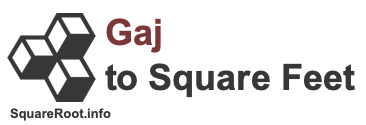1 gaj in square feetHere we will explain and show you how to convert 1 gaj to square feet. Before we continue, note that 1 gaj to square feet is the same as 1 gaj to sq ft, 1 gaj to ft2, and 1 gaj to ft².

Gaj and square feet are both measurements of an area, like a plot of land. One gaj is equal to 8.913592398096 square feet. Therefore, we can make the following gaj to square feet formula:

gaj × 8.913592398096
= square feet

Below is the math using our gaj to square feet formula to get 1 gaj in square feet.

= gaj × 8.913592398096
= 1 × 8.913592398096
= 8.913592398096
1 gaj ≈ 8.91 square feet

We have seen real estate brokers and others rounding up a gaj to nine square feet. Consequently, they simply multiply 1 gaj by nine to get it converted to square feet. In that case, this is how you would convert 1 gaj to square feet:

gaj × 9 = square feet
1 × 9 = 9
1 gaj = 9 square feet

Gaj to Square Feet Converter
Please enter another gaj area in the box below to have it converted to square feet.

Convert  gaj to square feet.

2 gaj in square feet
Here is the next area in gaj on our list that we have converted to square feet for you.